# JP2013127750A - Partitioning device, method and program - Google Patents

## Info

Publication number
JP2013127750A
JP2013127750A JP2011277623A JP2011277623A JP2013127750A JP 2013127750 A JP2013127750 A JP 2013127750A JP 2011277623 A JP2011277623 A JP 2011277623A JP 2011277623 A JP2011277623 A JP 2011277623A JP 2013127750 A JP2013127750 A JP 2013127750A
Authority
JP
Japan
Prior art keywords
partition
partitioning
cost
division
boundary line
Prior art date
Legal status (The legal status is an assumption and is not a legal conclusion. Google has not performed a legal analysis and makes no representation as to the accuracy of the status listed.)
Pending
Application number
JP2011277623A
Other languages
Japanese (ja)
Inventor

Harushio Hidaka

Original Assignee
Nippon Telegr & Teleph Corp <Ntt>

Priority date (The priority date is an assumption and is not a legal conclusion. Google has not performed a legal analysis and makes no representation as to the accuracy of the date listed.)
Filing date
Publication date
Application filed by Nippon Telegr & Teleph Corp <Ntt>, 日本電信電話株式会社 filed Critical Nippon Telegr & Teleph Corp <Ntt>
Priority to JP2011277623A priority Critical patent/JP2013127750A/en
Publication of JP2013127750A publication Critical patent/JP2013127750A/en
Pending legal-status Critical Current

• 238000000638 solvent extraction Methods 0.000 title claims abstract description 56
• 238000005192 partition Methods 0.000 claims abstract description 169
• 238000000034 method Methods 0.000 claims abstract description 25
• 230000001174 ascending Effects 0.000 claims abstract description 5
• 241001522296 Erithacus rubecula Species 0.000 claims description 7
• 238000011156 evaluation Methods 0.000 claims description 3
• 230000004044 response Effects 0.000 abstract description 18
• 230000003247 decreasing Effects 0.000 description 2
• 230000000694 effects Effects 0.000 description 2
• 238000010586 diagram Methods 0.000 description 1
• 230000004048 modification Effects 0.000 description 1
• 238000006011 modification reaction Methods 0.000 description 1
• 230000011218 segmentation Effects 0.000 description 1
• 230000001629 suppression Effects 0.000 description 1

## Images

•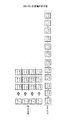•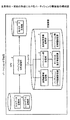•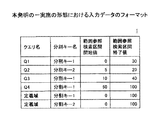•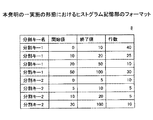•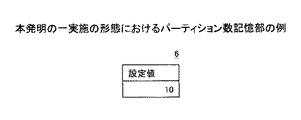•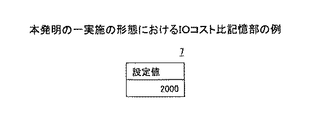•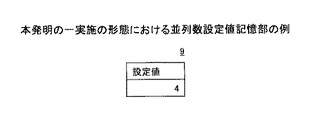•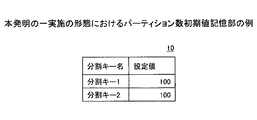•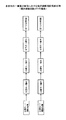••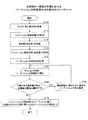•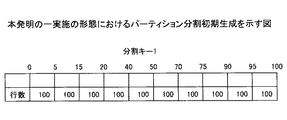•••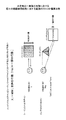•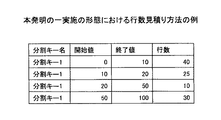•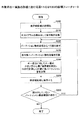•••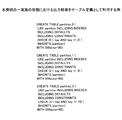## Abstract

PROBLEM TO BE SOLVED: To perform appropriate partition division for the purpose of improving response performance of range-referenced parallel access for a set number of partitions.
The present invention determines boundary lines in ascending order of key values for key items of input data, and sets each partition to the number of rows obtained by dividing the acquired predetermined number of rows by a predetermined initial number of partitions. Divide and store each divided partition candidate. Boundary lines that can be deleted by deleting one boundary line, generating partitioning, obtaining the number of parallel scan processes, and using the maximum IO cost assigned to each thread as the IO cost for the query of input data This is performed until there is no more partition, and the partition division with the smallest cost value equal to or less than the predetermined number of partitions is output from the partition division candidates.
[Selection] Figure 2

## Description

The present invention relates to a partitioning apparatus, method, and program, and in particular, when handling a large data size in a database, the search target data is distributed by partitioning a table, and a response is obtained by simultaneously accessing in parallel. The present invention relates to a table partitioning apparatus, method, and program for improving performance.
In commercial databases, there is a design advisor that recommends whether or not partitioning is necessary for a given workload and supports performance improvement and database design (for example, see Non-Patent Documents 1 and 2). In this way, designing a database with a large data size is very complicated, and is itself a field that requires tools support and an automatic partitioning method.
However, the conventional technology recommends whether or not partitioning should be performed, and supports the output of the partitioning method (the value of the partitioning key that specifies the boundary) when performing partitioning specifically. Absent.
On the other hand, the end point of the range reference search section of the input data is extracted and stored as a boundary candidate in the boundary line candidate storage means, and partitioning with the boundary line candidate and the IO cost required for data access is generated is generated. Then, one boundary line excluding both ends of the range reference search section is deleted, and the partition partition after the deletion is generated. Then, the IO cost increment value after the boundary line is deleted is calculated, and the partition division candidate whose partition division candidate number is equal to or less than the predetermined number N and whose IO cost increment value is the smallest is output as the partition division result. A range reference search is possible. However, since parallel access is not taken into consideration, the effect cannot be expected as a partitioning technique for improving response performance of parallel access.
Oracle Database Performance Tuning Guide 11g Release (11.1) 18 SQL Access Advisor http://otndnld.oracle.com/document/products/oracle11g/111/doc_dvd/server.111/E0574302/advisor.htm Using DB2 V8.2 Design Advisor Part 2: Help information for designing a data warehouse http://www-06.ibm.com/jp/domino01/mkt/dminfo.nsf/499721c3388537bd49256b1a001aab28/4925722f004efcee492570d4005a65ef/\$ FILE / Using% 20the% 20DB2% 20V8.pdf
However, in the above-mentioned range reference search, when executing parallel access by partitioning a table, the response time is the maximum response time among a plurality of parallel access processing units (hereinafter referred to as “threads”). Therefore, if the search target data is not evenly distributed to each partition, the effect of parallel access cannot be enjoyed. Specifically, if the number of parallel accesses (typically equal to the number of CPU cores) is 4, for example, if the response time of the remaining 1 thread does not change even if the response time of 3 threads is reduced, the system The overall response performance does not change.
When considering a partitioning method for improving the response performance of parallel access, reduce the response time of the thread that maximizes the response time when the search target data is distributed in each partition and accessed simultaneously in parallel. By suppressing it, the response performance of the entire system can be improved. Therefore, the search target data is distributed to each thread so that the IO cost shared by each thread is equal. Therefore, the number of partitions (for example, the number of partitions is assumed to be 12) is associated with the number of parallel accesses (for example, the number of parallel accesses is 4), and the access destination partition in charge of each thread is associated by round robin ( In practice, the process of determining the access destination for parallel access depends on the implementation of the parallel access process), and the search target data is distributed to each partition so that the IO cost handled by each thread becomes equal. An example of this is shown in FIG. This figure shows a case in which the number of parallel accesses is a set value (for example, four, the number of CPU cores) and the association of parallel access units (hereinafter referred to as “threads”) to access destination partitions is determined by round robin. FIG. 1 shows an example in which a thread i is associated with a partition (i mod 4), but actually depends on the implementation of parallel access. Here, the IO cost is defined as the sum of contributions from all queries in all partitions and workloads. If the IO cost of partition i for query Q in the workload is X j , the cost of the system is
Σ Q max (X 0 + X 4 + X 8 +…, X 1 + X 5 + X 9 +…, X 2 + X 6 + X 10 +…, X 3 + X 7 + X 11 +…)
It becomes. However, X i = min [(1 + p) × number of target rows of partition i, total number of rows of partition i], and 1 + p is IO cost per row of index scan / IO cost per row of sequence scan It is. For each partition, an index scan or a sequential scan is selected with the smaller IO cost. The number of rows is estimated by linear interpolation from a histogram of the number of rows distribution.
As described above, one of the distribution methods that equalizes the IO cost handled by each thread is to subdivide each row as each partition and maximize the number of partitions. However, if the number of partitions is increased too much, the overhead required for the process of determining the access destination partition and the unique process for each partition becomes so large that it cannot be ignored. As a result, in the partitioning for the purpose of improving the response performance, the number of partitions must be a finite value.
The present invention has been made in view of the above points, and provides a partitioning device, method, and program capable of performing appropriate partitioning for the purpose of improving the response performance of range-referenced parallel access with respect to the set number of partitions. The purpose is to provide.
In order to solve the above problem, the present invention (Claim 1) is a partitioning device for partitioning a database table for parallel processing,
Boundary line candidate storage means is initialized, boundary lines are determined in ascending order of key values for the key items of the input data, and each partition is divided into the number of lines obtained by dividing the acquired predetermined number of lines by the predetermined initial number of partitions. Partition initial division means for equally dividing, storing the boundary lines in the boundary line candidate storage means, and storing each divided partition division candidate in the partition division candidate storage means;
Delete one of the partitioning boundaries except for both ends, generate the partitioning after deletion, obtain the parallel number of parallel scan processing, and assign it to each parallel scan processing unit (thread) Boundary line deletion means for storing the maximum value among IO costs as the IO cost for the query of the input data and storing in the partition candidate storage means,
Evaluation means for outputting a partition division having a minimum cost value with the number of partitions equal to or less than a predetermined number of partitions from the partition division candidates of the partition division candidate storage means.
In the present invention (Claim 2), in the boundary line deletion unit, each partition is associated with the parallel number of threads in a round robin manner.
Further, according to the present invention (Claim 3), in the boundary line deletion means, the IO cost is calculated using a ratio of an IO cost of index scan and a sequential scan IO cost per given row.
According to the present invention, by using input data and partitioning so that the access cost in parallel access is more evenly distributed to each thread for a given workload, parallel access for range reference search can be performed. Response performance can be improved. In addition, it is possible to realize an advisor that outputs an appropriate partition partitioning method (partition key value for designating a boundary line) so that the distribution of the access cost of each thread is even.
It is a figure which shows the definition of IO cost. It is a block diagram of the partition dividing apparatus in one embodiment of this invention. It is a format of input data in one embodiment of the present invention. It is a format of the histogram memory | storage part in one embodiment of this invention. It is an example of the partition number memory | storage part in one embodiment of this invention. It is an example of the IO cost ratio memory | storage part in one embodiment of this invention. It is an example of the parallel number setting value memory | storage part in one embodiment of this invention. It is an example of the partition number initial value storage part in one embodiment of this invention. It is an example (when the number of boundary line candidates is 3) of the boundary line candidate memory | storage part in one embodiment of this invention. It is an example of the partition division candidate memory | storage part in one embodiment of this invention. It is a flowchart of the whole operation | movement of the partition dividing apparatus in one embodiment of this invention. It is a figure which shows the partition division initial generation in one embodiment of this invention. It is an example of an output result of partitioning in an embodiment of the present invention. It is an example of calculation of IO cost value in one embodiment of the present invention. It is an example of individual IO cost value calculation with respect to each range reference search in an embodiment of the present invention. It is an example of the line number estimation method in one embodiment of this invention. It is a flowchart of the initial generation process of partition division of S130 of FIG. 11 in one embodiment of this invention. It is a detailed flowchart of S160 of FIG. 11 in one embodiment of this invention. FIG. 19 is a detailed flowchart of S350 in FIG. 18 according to an embodiment of the present invention. It is an example which utilizes the output result in one embodiment of this invention as a table definition.
Embodiments of the present invention will be described below with reference to the drawings.
First, the outline of the present invention will be described.
The present invention will be specifically described below.
FIG. 2 shows the configuration of the partitioning device in one embodiment of the present invention.
The partition division apparatus shown in FIG. 1 includes an input data storage unit 1, a partition division processing unit 2, an output data storage unit 3, a boundary line candidate storage unit 4, a partition division candidate storage unit 5, a partition number storage unit 6, and an IO cost ratio. The set value storage unit 7, the histogram storage unit 8, the parallel number set value storage unit 9, and the partition number initial value storage unit 10 are configured.
In the figure, the input data storage unit 1, the output data storage unit 3, the boundary line candidate storage unit 4, the partition division candidate storage unit 5, the partition number storage unit 6, the IO cost ratio setting value storage unit 7, and the histogram storage unit. 8, the parallel number set value storage unit 9 and the partition number initial value storage unit 10 are shown to be stored in different storage media, but are not limited to this example. You may store in the storage medium which stores several types of data, such as a database.
The partition division processing unit 2 is a processing unit that operates on a CPU, and is connected to the storage unit via a data bus.
FIG. 3 shows a format of input data according to the embodiment of the present invention. The input data includes a query name, a split key name, a range reference search interval start value, and a range reference search interval end value. As shown in the figure, the input data also registers the range (definition area) that the split key can take. When the input data is input, it is stored in the input data storage unit 1.
FIG. 4 shows the format of the histogram storage unit according to the embodiment of the present invention. The histogram storage unit 8 stores the division key name, the start value, the end value, and the number of rows.
FIG. 5 shows an example of the partition number storage unit according to the embodiment of the present invention. The partition storage unit 6 stores a partition number setting value N in advance.
FIG. 6 shows an example of the IO cost ratio storage unit according to the embodiment of the present invention. The IO cost ratio storage unit 7 stores the IO cost of index scan per row and the IO of sequential scan set by the operator. Stores the cost ratio. For example, the IO cost ratio p (set value) in step 100 of FIG. 11 is held. For example, when p = 2000, “2000” is held.
The data in the partition number storage unit 6 and the IO cost ratio setting value storage unit 7 are used for the calculation of the IO cost increment value by the partition division processing unit 2.
FIG. 7 is an example of the parallel number set value storage unit in the embodiment of the present invention, in which the parallel number is stored in advance.
FIG. 8 is an example of the partition number initial value storage unit according to the embodiment of the present invention, and stores the initial value of the partition number for each division key.
FIG. 9 is an example of the boundary line candidate storage unit in the embodiment of the present invention, and shows a case where the number of boundary line candidates is three. Addition and deletion of the split key are performed by inserting and deleting at a position where the split key value maintains the ascending order.
FIG. 10 is an example of a partition candidate storage unit according to an embodiment of the present invention. FIG. 10 (a) shows an example of initial generation, and FIG. 10 (b) shows an example other than the initial generation. Show. In FIG. 5A, if the number of boundary lines of the division key 1 is 3, and the number of boundary lines of the division key 2 is 3, the number of partitions is (3-1) × (3-1) = 4.
The operation of the apparatus having the above configuration will be described.
FIG. 11 is a flowchart of the overall operation of the partitioning device according to one embodiment of the present invention. In the following, it is assumed that input data has already been input and stored in the input data storage unit 1.
Step 100) The partition division processing unit 2 acquires the IO cost ratio p from the IO cost ratio set value storage unit 7.
Step 110) The partition division processing unit 2 acquires the partition number N from the partition number storage unit 6.
Step 120) The partition division processing unit 2 acquires the parallel number from the parallel number set value storage unit 9.
Step 125) The partition division processing unit 2 acquires an initial value of the number of partitions from the partition number initial value storage unit 10.
Step 130) The partition division processing unit 2 performs initial generation of partition division.
When partitioning is performed, first, partition partitioning with the same number of rows of search target data is generated as initial partition generation (the initial partition partition number is set). An example of this is shown in FIG. In the example of initial partition division generation shown in the figure, the partition candidate is divided into equal numbers by the number of rows so that the number of partitions becomes the initial number of partitions (setting value, for example, 10) as the initial candidate. .
When partitioning is performed, the input data shown in FIG. 3 is read from the input data storage unit 1, the most divided partition partition is generated based on the input data, and stored in the boundary line candidate storage unit 4. Specifically, as shown in FIG. 9, all the end points (start value, end value) of the range reference search range of the input data are used, and partition division is obtained using this as a boundary line. Thereby, a division key boundary candidate as shown in FIG. 10A is obtained. In the example shown in the figure, if the number of boundary lines of “partition key 1” is 3, and the number of boundary lines of “partition key 2” is 3, the number of partitions = (3-1) × (3-1) = 4. .
Step 140) The partition division processing unit 2 stores the set of division keys in the partition division candidate storage unit 5 as partition division candidates as shown in FIG. The number of partitions can be derived from FIG.
Step 150) The partitioning processing unit 2 determines whether there is a boundary that can be deleted. Note that both ends of the definition area cannot be deleted. Specifically, if the number of partitions> the set value N, it is determined that it exists, and the process proceeds to step 160. If the number of partitions ≦ the set value N, it is determined that it does not exist, and the process proceeds to step 170.
Step 160) When it is determined that there is a boundary line that can be deleted in Step 150, the partition division when the number of boundary lines is decreased by 1 is acquired, stored in the partition division candidate storage unit 5, and the process returns to Step 140. Detailed processing will be described later with reference to FIG.
Step 170) The partition division processing unit 2 deletes the boundary line as described above, thereby increasing the access range, generating partition division, calculating the IO cost increment value, and satisfying the number of partitions ≦ N from the partition division candidates. The partition division with the smallest IO cost value is output to the output data storage unit 3. The result of partitioning the input data is output as shown in FIG.
Next, the partition generation initial generation process in step 130 will be described.
FIG. 17 is a flowchart of the partition generation initial generation process in step 130 of FIG. 11 according to the embodiment of this invention.
Step 200) The partition division processing unit 2 initializes the boundary line candidates in the boundary line candidate storage unit 4.
Step 210) The histogram is read from the histogram storage unit 8 to obtain the total number of rows.
Step 220) One row is read from the partition number initial value in the partition candidate storage unit 5.
Step 230) Based on the total number of rows acquired in step 210 and the initial number of partitions read in step 220, the total number of rows divided by the initial number of partitions is calculated.
Step 240) Boundaries are determined in order from the smallest key value for the key item, and each partition is equally divided into the number of rows calculated in Step 230.
Step 250) The partitions equally divided in Step 240 are stored in the boundary line candidate storage unit 4. When dividing a partition, the input data shown in FIG. 3 is read from the input data storage unit 1, the most divided partition division is generated based on the input data, and a set of division key values is used as a boundary candidate. Stored in the line candidate storage unit 4. Specifically, as shown in FIG. 9, all the end points (start value, end value) of the range reference search range of the input data are used, and partition division is obtained using this as a boundary line. Thereby, a division key boundary candidate as shown in FIG. 10A is obtained. In the example shown in the figure, if the number of boundary lines of “partition key 1” is 3, and the number of boundary lines of “partition key 2” is 3, the number of partitions = (3-1) × (3-1) = 4. .
Step 260) If there is an initial value of the number of partitions that has not been read, the process returns to Step 220. If all are read, the process is terminated.
Next, the partition division acquisition process when the number of boundary lines in step 160 in FIG. 11 is decreased by 1 will be described.
FIG. 18 is a detailed flowchart of step 160 in FIG. 11 according to the embodiment of the present invention.
Step 300) When the number M of boundary lines is input, the partition division processing unit 2 determines the number of boundary lines after deleting one boundary line in the partition division candidate storage unit 5 when there is one boundary line that can be deleted. M is written, and the partition division candidates having the number M of boundary lines in the partition division candidate storage unit 5 are initialized.
Step 310) From the partition division candidates having the number M + 1 of the boundary lines in the partition division candidate storage unit 5, one partition division having the smallest IO cost is selected.
Step 315) If the number of partitions of the selected partition division is equal to or less than the number of partitions N acquired from the partition number storage unit 6 (the number of partitions of the selected partition division ≦ N), the process proceeds to Step 395. Otherwise, the process proceeds to step 320.
Step 320) One boundary line (however, both ends of the domain are excluded) is selected from the partition division candidates in the partition division candidate storage unit 5.
Step 330) The IO cost value of a memory (not shown) is initialized to zero.
Step 340) Read one line of input data from the input data storage unit 1 and take out a range reference search section.
Step 350) The IO cost for the range reference search of the input data is calculated and added to the IO cost value of the memory (not shown). Details will be described later with reference to FIG.
Step 370) If there is data that has not been read from the input data storage unit 1, the process proceeds to Step 340.
Step 380) The partition division candidate is additionally stored in the partition division candidate storage unit 5.
Step 390) If there is an unselected boundary line in the partition candidate storage unit 5, the process proceeds to Step 320.
Step 395) If there is an unselected partition division candidate M + 1 with a minimum IO cost in the partition division candidate storage unit 5, the process proceeds to Step 310.
Next, the IO cost calculation processing for the input data range reference search in step 350 of FIG. 18 will be described.
FIG. 19 is a detailed flowchart of step 350 in FIG. 18 according to an embodiment of the present invention.
Step 400) As shown in FIG. 1, the partition division processing unit 2 associates each partition with the number of parallel threads in round robin. When the number of parallel accesses shown in FIG. 1 is a set value (for example, 4 and the number of CPU cores) and the thread access is determined by round robin,
max (X 0 + X 4 + X 8 + ..., X 1 + X 5 + X 9 + ..., X 2 + X 6 + X 10 + ..., X 3 + X 7 + X 11 + ...)
And However, X i = min [(1 + p) × number of target rows of partition i, total number of rows of partition i].
Step 410) The following IO cost calculation is performed and added to the IO cost value.
In the calculation of the IO cost value, as shown in FIG. 14, the sum of contributions from all the range reference searches of input data and IO cost values over all partitions is used. Here, as shown in FIG. 15, the individual IO cost value for each range reference search includes the estimated value of the number of rows and the ratio of the IO cost of the index scan per row to the IO cost of the sequential scan (system by the operator). Calculated based on the smaller of the following values calculated based on the setting value):
-IO cost of index scan per row x number of target rows;
-IO cost of sequential scan per row x number of rows in the partition;
However, for the number of rows, an estimated value using the histogram shown in FIG. 4 of the histogram storage unit 8 is used. In the example of FIG. 16, in order to estimate the number of rows where the value of the split key 1 is between 15 and 40, linear interpolation is used.
Estimated number of rows = FLOOR [25 x (20-15) / (20-10) + 10 x (40-20) / (50-20)]
However, FLOOR represents rounding down after the decimal point. In this way, partition partitioning is generated until the number of partitions reaches the set value or until there is no boundary that can be deleted (both ends of the definition area are deleted loads), and after evaluating the IO cost value, the partition among the partition partition candidates The partition division result that minimizes the IO cost value satisfying the condition that the number satisfies the set value or less is output.
As shown in the flowchart above, when partitioning is performed, first, partition partitioning is performed in which the number of rows of search target data is uniform (initially generated partition partitioning number is a set value). An example of this is shown in FIG. In the example of initial partition division generation shown in the figure, an initial candidate is a partition division in which the domain of the partition key is equally divided by the number of rows so that the number of divisions becomes the initial value of the number of partitions.
Next, the process of deleting one boundary line is executed, all possible partition divisions are generated, and the IO cost is calculated. In this process, the IO cost value is evaluated over each candidate. In the calculation of the IO cost value, as shown in FIG. 14, the sum of contributions from all the range reference searches of input data and IO cost values over all partitions is used. In this way, partitioning is generated until the number of partitions reaches the set value or until there is no boundary that can be deleted (both ends of the definition area cannot be deleted), and after evaluating the IO cost value, the partition among the partitioning candidates The partition division result that minimizes the IO cost value satisfying the condition that the number satisfies the set value or less is output.
The result of the above-described processing can be used for defining a partition table, for example. FIG. 20 shows an example in which the output result is used as a table definition. The figure shows the case of 3 partitions. This is an example in which the boundary line is key = 2.5 with respect to the domain 0 <key ≦ 10 of the value of the partitioning key key for partitioning the table partition into the tables partition 0 , partition 1 , and partition 2 .
As described above, for the input data workload, partitions are allocated in a round-robin fashion so that the access cost for parallel access is more evenly distributed to each thread, and the size of each allocated thread is assigned to each thread. The IO cost is calculated, the maximum value of the IO cost of each thread is set as the cost of the entire system, and among the partition partition candidates, the condition that the number of partitions is a predetermined number or less is satisfied so that the cost of the entire system is minimized. Perform partitioning that minimizes IO cost. This is because the overall response time is determined solely by the response time for the thread with the largest access cost, so partitioning with uneven access cost distribution to threads is not appropriate partitioning for the purpose of improving response performance. Because there is no.
Note that the processing of the partition division processing unit 2 in the above-described embodiment can be constructed as a program, installed in a computer used as a partition division apparatus, executed, or distributed via a network.
The present invention is not limited to the above-described embodiments, and various modifications and applications are possible within the scope of the claims.
DESCRIPTION OF SYMBOLS 1 Input data memory | storage part 2 Partition division | segmentation process part 3 Output data memory | storage part 4 Boundary line candidate memory | storage part 5 Partition division candidate memory | storage part 6 Partition number memory | storage part 7 IO cost ratio setting value memory | storage part 8 Histogram memory | storage part 9 Parallel number setting value memory | storage Section 10 Initial partition number storage section

## Claims (7)

1. A partitioning device for partitioning a database table for parallel processing,
Boundary line candidate storage means is initialized, boundary lines are determined in ascending order of key values for the key items of the input data, and each partition is divided into the number of lines obtained by dividing the acquired predetermined number of lines by the predetermined initial number of partitions. Partition initial division means for equally dividing, storing the boundary lines in the boundary line candidate storage means, and storing each divided partition division candidate in the partition division candidate storage means;
Delete one of the partitioning boundaries except for both ends, generate the partitioning after deletion, obtain the parallel number of parallel scan processing, and assign it to each parallel scan processing unit (thread) Boundary line deletion means for storing the maximum value among IO costs as the IO cost for the query of the input data and storing in the partition candidate storage means,
An evaluation unit that outputs a partition division that minimizes a cost value that is equal to or less than a predetermined number of partitions from the partition division candidates of the partition division candidate storage unit;
A partitioning device characterized by comprising:
2. The boundary line deleting means is
The partition dividing apparatus according to claim 1, wherein each partition is associated with the parallel number of threads in round robin.
3. The boundary line deleting means is
2. The partitioning device according to claim 1, wherein the IO cost is calculated using a ratio of an IO cost of an index scan per a given row and an IO cost of a sequential scan.
4. A partitioning method for partitioning a database table for parallel processing,
The partition initial division means initializes the boundary line candidate storage means, determines the boundary lines in ascending order of key values for the key items of the input data, and divides the obtained predetermined number of rows by the predetermined initial number of partitions. Partition initial division step of equally dividing each partition into the number of rows, storing the boundary line in the boundary line candidate storage unit, and storing each divided partition division candidate in the partition division candidate storage unit;
The boundary line deletion means deletes one boundary line except for both ends from among the partition line boundaries, generates a partition partition after deletion, obtains the number of parallel scan processes, and acquires the parallel scan processing unit (thread ) A boundary line deletion step in which the maximum value among the IO costs assigned to each input is set as the IO cost for the query of the input data, and the process of storing in the partitioning candidate storage unit is performed until there is no boundary line that can be deleted;
An evaluation unit that outputs a partition division that minimizes a cost value that is equal to or less than a predetermined number of partitions from the partition division candidates of the partition division candidate storage unit;
Partitioning method characterized in that:
5. In the boundary deletion step,
5. The partitioning method according to claim 4, wherein each partition is associated with the parallel number of threads in round robin.
6. In the boundary deletion step,
5. The partitioning method according to claim 4, wherein the IO cost is calculated using a ratio of an IO cost of index scan and a sequential scan per given row.
7. Computer
A partitioning program for causing each of the means of the partitioning device according to any one of claims 1 to 3 to function.
JP2011277623A 2011-12-19 2011-12-19 Partitioning device, method and program Pending JP2013127750A (en)

## Priority Applications (1)

Application Number Priority Date Filing Date Title
JP2011277623A JP2013127750A (en) 2011-12-19 2011-12-19 Partitioning device, method and program

## Applications Claiming Priority (1)

Application Number Priority Date Filing Date Title
JP2011277623A JP2013127750A (en) 2011-12-19 2011-12-19 Partitioning device, method and program

## Publications (1)

Publication Number Publication Date
JP2013127750A true JP2013127750A (en) 2013-06-27

# Family

## Family Applications (1)

Application Number Title Priority Date Filing Date
JP2011277623A Pending JP2013127750A (en) 2011-12-19 2011-12-19 Partitioning device, method and program

## Country Status (1)

JP (1) JP2013127750A (en)

## Cited By (1)

* Cited by examiner, † Cited by third party
Publication number Priority date Publication date Assignee Title
KR101833996B1 (en) 2017-08-18 2018-04-13 주식회사 마크베이스 System and method for searching data

## Cited By (1)

* Cited by examiner, † Cited by third party
Publication number Priority date Publication date Assignee Title
KR101833996B1 (en) 2017-08-18 2018-04-13 주식회사 마크베이스 System and method for searching data

## Similar Documents

Publication Publication Date Title
JP2019194882A (en) Mounting of semi-structure data as first class database element
US10915528B2 (en) Pluggable storage system for parallel query engines
US9672235B2 (en) Method and system for dynamically partitioning very large database indices on write-once tables
US20190018872A1 (en) Early exit from table scans of loosely ordered and/or grouped relations using nearly ordered maps
US9805077B2 (en) Method and system for optimizing data access in a database using multi-class objects
JP2019530068A (en) Table incremental clustering maintenance
EP3014488B1 (en) Incremental maintenance of range-partitioned statistics for query optimization
US20070174278A1 (en) Method and system for performing logical partial declustering
US10831709B2 (en) Pluggable storage system for parallel query engines across non-native file systems
US20190278773A1 (en) Grouping in analytical databases
Cahsai et al. Scaling k-nearest neighbours queries (the right way)
JPWO2012114402A1 (en) Database management apparatus and database management method
US9292554B2 (en) Thin database indexing
US11074242B2 (en) Bulk data insertion in analytical databases
JP2013127750A (en) Partitioning device, method and program
JP2013080403A (en) Table partitioning device, method, and program
US10877973B2 (en) Method for efficient one-to-one join
JP2007048318A (en) Relational database processing method and relational database processor
US9292553B2 (en) Queries for thin database indexing
KR102005343B1 (en) Partitioned space based spatial data object query processing apparatus and method, storage media storing the same
Naeem et al. Optimising HYBRIDJOIN to Process Semi-Stream Data in Near-real-time Data Warehousing.
Watari et al. Efficient Aggregation Query Processing for Large-Scale Multidimensional Data by Combining RDB and KVS
Zhou et al. WACO: Workload Aware Column Order for Scan Operator in Wide Table
Latchoumi et al. Efficient Query Optimization Techniques in Data Warehouses

## Legal Events

Date Code Title Description
RD02 Notification of acceptance of power of attorney

Free format text: JAPANESE INTERMEDIATE CODE: A7422

Effective date: 20131004